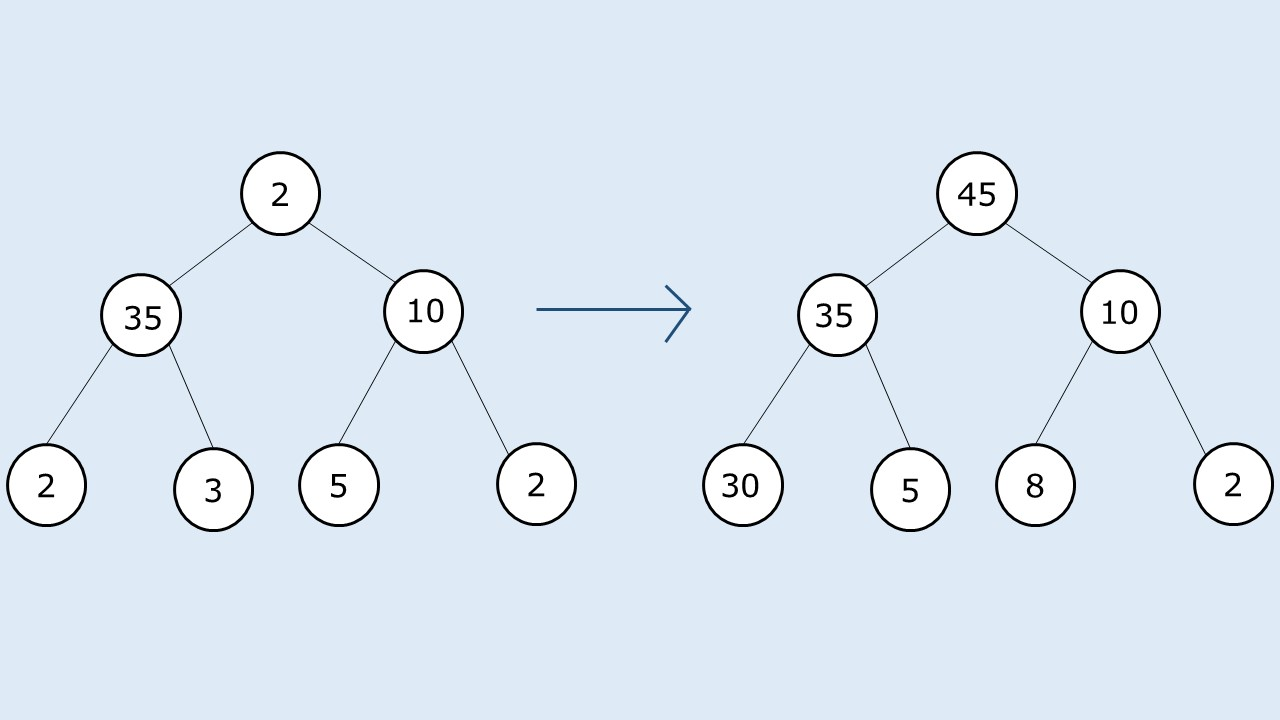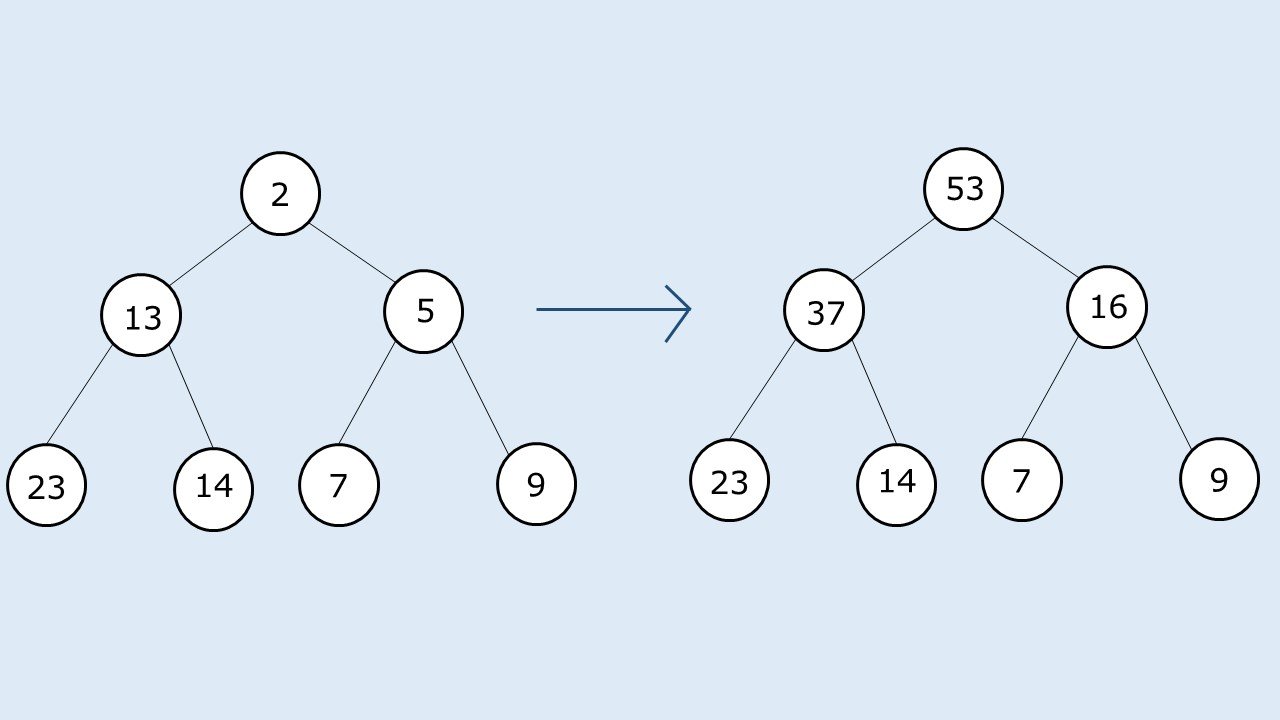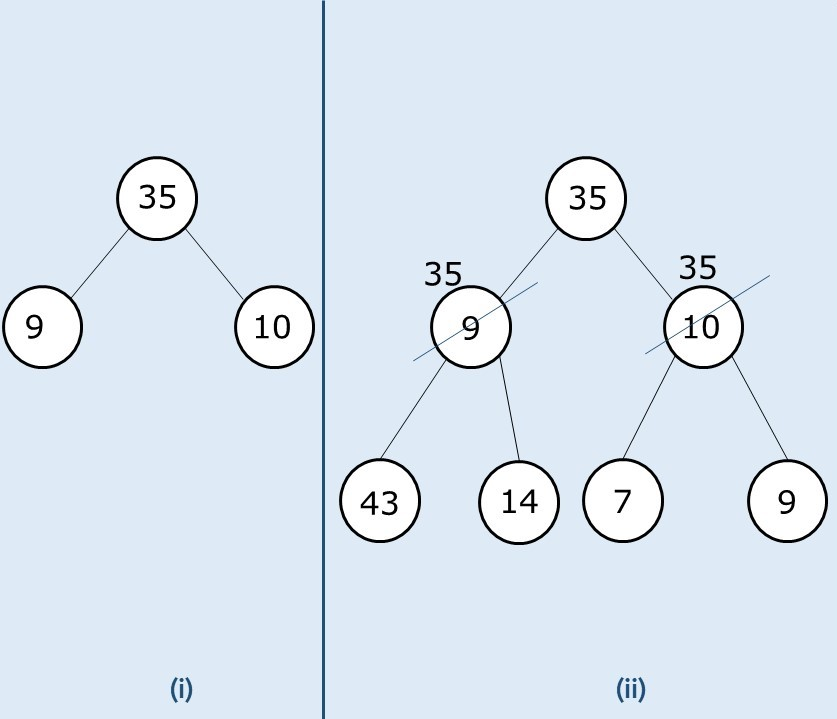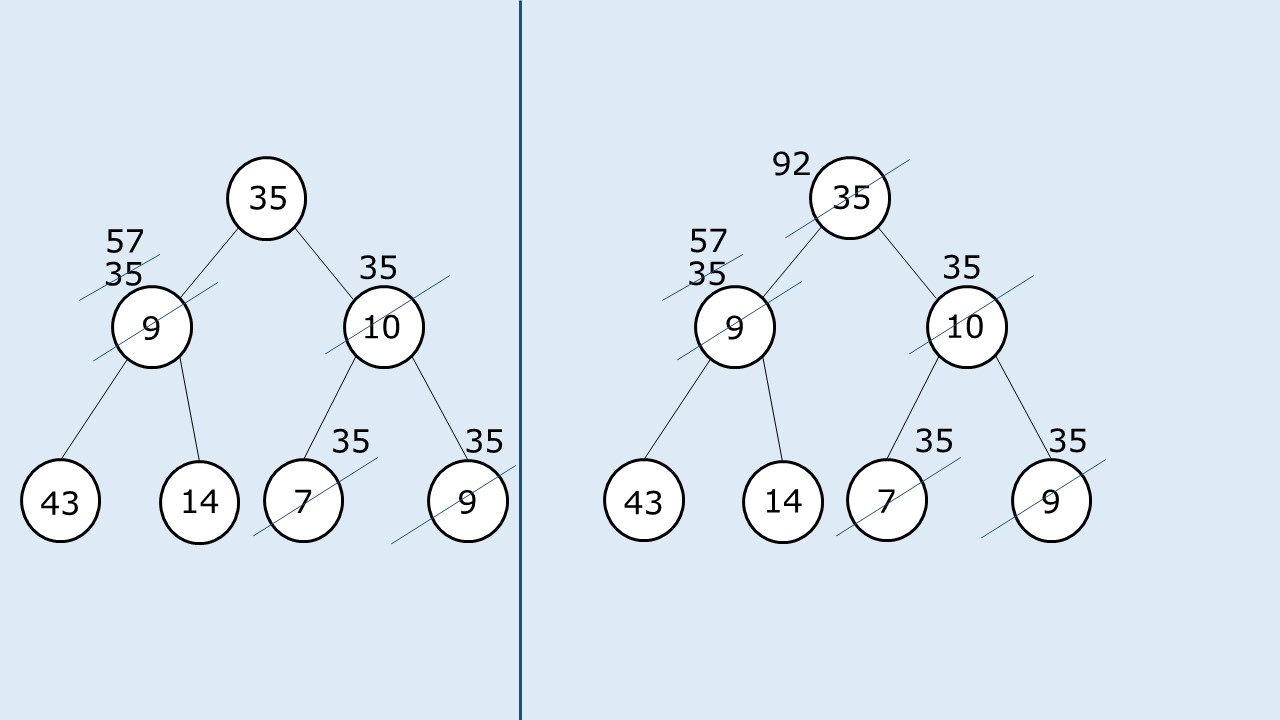# Check for Children Sum Property in a Binary Tree

Problem Statement: Children Sum Property in a Binary Tree. Write a program that converts any binary tree to one that follows the children sum property.

The children sum property is defined as, For every node of the tree, the value of a node is equal to the sum of values of its children(left child and right child).

Note:

• The node values can be increased by 1 any number of times but decrement of any node value is not allowed.
• A value for a NULL node can be assumed as 0.
• You are not allowed to change the structure of the given binary tree.
```Example:```

Pre-req: Tree Traversals

Disclaimer: Don’t jump directly to the solution, try it out yourself first.

### Solution :

Intuition:

The first intuition that comes to our mind is to do a tree traversal and at every node make the node value equal to the sum of value(s) of its children. This approach will work in a tree-like this(given below), if we do a postorder traversal and update the node values after visiting the children.As in the given tree, every node value is less than the sum of the node values of its children; this approach works. Now we will see where this approach will fail and why. If we consider the following case:• In case (i), we see that the root node’s value is greater than the sum of both the leaf nodes. In such a case it is not possible to assign a node as the sum of its children( as we are not allowed to decrement a value). In such a case we simply assign the node value of the children to its parent.
• In case (ii) as we have a tree further down, we first implement the strategy discussed in case(i), now we see that at the second level we are having both the cases where, 35 is less than (43+14) and 35 is greater than (7+9). For the latter case we again implement the strategy and make both its children 35. For the former case, we simply traverse the tree and set it to the sum of its children.Approach:

We perform a tree traversal and check whether the current node value is greater than the sum of node values of its children. If this is the case, we simply assign its children to the same value of the current node and then recurse for the children. We do so because we are not allowed to decrement a node value. So we set the children to a large value in order to increment the parent.

It can happen in subsequent recursions that this child value is further changed, therefore it is necessary that when we return to a node after returning from its children, we set it to the sum of node values of its children explicitly.

The algorithm approach can be stated as follows:

• We perform a simple dfs traversal on the tree.
• For the base case, if the node is pointing to NULL, we simply return.
• At every node, first we find the sum of values of the children( For a NULL child, value is assumed to be 0).
• If node’s value > sum of children node value, we assign both the children’s value to their parent’s node value.
• Then we visit the children using recursion.
• After we return to the node after visiting its children, we explicitly set its value to be equal to the sum of its values of its children.

Dry Run: In case you want to watch the dry run for this approach, please watch the video attached below.

Code:

## C++ Code

``````#include <bits/stdc++.h>

using namespace std;

struct node {
int data;
struct node * left, * right;
};

void reorder(node * root) {
if (root == NULL) return;
int child = 0;
if (root -> left) {
child += root -> left -> data;
}
if (root -> right) {
child += root -> right -> data;
}

if (child < root -> data) {
if (root -> left) root -> left -> data = root -> data;
else if (root -> right) root -> right -> data = root -> data;
}

reorder(root -> left);
reorder(root -> right);

int tot = 0;
if (root -> left) tot += root -> left -> data;
if (root -> right) tot += root -> right -> data;
if (root -> left || root -> right) root -> data = tot;
}
void changeTree(node * root) {
reorder(root);
}

struct node * newNode(int data) {
struct node * node = (struct node * ) malloc(sizeof(struct node));
node -> data = data;
node -> left = NULL;
node -> right = NULL;

return (node);
}

int main() {

struct node * root = newNode(2);
root -> left = newNode(35);
root -> left -> left = newNode(2);
root -> left -> right = newNode(3);
root -> right = newNode(10);
root -> right -> left = newNode(5);
root -> right -> right = newNode(2);

changeTree(root);

return 0;
}
``````

Time Complexity: O(N)

Reason: We are doing a simple tree traversal.

Space Complexity: O(N)

Reason: In the worst case( skewed tree), space complexity can be O(N).

## Java Code

``````import java.util.*;
class Node {
int data;
Node  left,  right;
Node(int data)
{
this.data=data;
left=null;
right=null;
}
}
class TUF{
static void reorder(Node  root) {
if (root == null) return;
int child = 0;
if (root . left!=null) {
child += root . left . data;
}
if (root . right!=null) {
child += root . right . data;
}

if (child < root . data) {
if (root . left!=null) root . left . data = root . data;
else if (root . right!=null) root . right . data = root . data;
}

reorder(root . left);
reorder(root . right);

int tot = 0;
if (root . left!=null) tot += root . left . data;
if (root . right!=null) tot += root . right . data;
if (root . left!=null || root . right!=null) root . data = tot;
}
static void changeTree(Node  root) {
reorder(root);
}

public static void main(String args[]) {

Node  root = new Node(2);
root . left = new Node(35);
root . left . left = new Node(2);
root . left . right = new Node(3);
root . right = new Node(10);
root . right . left = new Node(5);
root . right . right = new Node(2);

changeTree(root);

}
}
``````

Time Complexity: O(N)

Reason: We are doing a simple tree traversal.

Space Complexity: O(N)

Reason: In the worst case( skewed tree), space complexity can be O(N).

Special thanks to Anshuman Sharma for contributing to this article on takeUforward. If you also wish to share your knowledge with the takeUforward fam, please check out this article